# mysql查看数据库大小以及使用情况?

1、进入information_schema 数据库(存放了其他的数据库的信息)

use information_schema;

2、查询所有数据的大小：

select concat(round(sum(data_length/1024/1024),2),'MB') as data from tables;

3、查看指定数据库的大小：

select concat(round(sum(data_length/1024/1024),2),'MB') as data from tables where table_schema='home';

4、查看指定数据库的某个表的大小

select concat(round(sum(data_length/1024/1024),2),'MB') as data from tables where table_schema='home' and table_name='members';information_schema是MySQL的系统数据库，information_schema里的tables表存放了整个数据库各个表的使用情况。

table_schema：数据库名

table_name：表名

table_rows：记录数

data_length：数据大小

index_length：索引大小

1、统计表使用空间

mysql> select concat(round(sum(data_length/1024/1024),2),'mb') as data from tables where table_schema='mydb' and table_name='mytable';

| data |

| 0.02mb |

1 row in set (0.00 sec)

2、统计数据库使用空间

mysql> select concat(round(sum(data_length/1024/1024),2),'MB') as data from tables where table_schema='mydb';

| data |

| 6.64MB |

1 row in set (0.00 sec)

3、统计所有数据使用空间

mysql> select concat(round(sum(data_length/1024/1024),2),'MB') as data from tables;

| data |

| 6.64MB |

1 row in set (0.01 sec)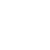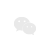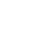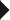免费拨打 400-1886560 或 0592-5580190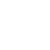返回顶部